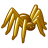Novice - Difference in Elevation variable used in if/elif statements

235
3
05-12-2018 01:42 PMNew Contributor

I took a Python class in 2012 and am attempting badly to use it for a project and I am stuck.  When I have explained what I want to do so far nobody understands what I am asking so I hope this comes through.

I have a hiking trail where I collected field data.  I converted the lines I collected to points, spaced one point every meter.  The first point (trailhead) has a value of 1 in the INDEX field (a field I created).  Every point after that point also has an INDEX value which increases from the previous point by a value of 1.  This INDEX per point will keep increasing by 1 until the last point on the trail has been reached.

I want to calculate the absolute value of the change in elevation between that first point (INDEX = 1) and every other point on the trail (INDEX = 2 to ???).  The end result will be, based on a pre-existing elevation field and an INDEX field, every single point will have a new value under a field called Diff_Elev_ft which is the change in elevation between the starting point and every other point.

Example

Elevation in ft for the point with an INDEX = 1 is 1,100 ft

Elevation in ft for a point further along the trail with INDEX = 100 is 1,300 ft

The Diff_Elev_ft between those points will be 200 ft, which will be entered into the Diff_Elev_ft field for the INDEX = 100 point

I hope this makes sense.  In my mind I need to set up the elevation from the INDEX = 1 point as a variable and for every other point (with an INDEX > 1) subtract that variable value (the elevation at the INDEX = 1 point) from all the other trail point elevations.  This is all based on a table which has an INDEX and Elev_ft value for each point on the trail.

Tags (3)
3 RepliesbyMVP Esteemed Contributor

Showing the code you are working with would help greatly.

Also remember Code Formatting... the basicsNew Contributor

Not really since I have none.  Since I am so new to Python I couldn't write a python script so I tried to do everything I needed to do for my project in model builder and was 2 steps from the end when I got stuck on this subtract every elevation from the starting elevation problem and I have been stuck for 3 days.  I am attempting to experiment with field calculator and am having no success at all.  No code.New Contributor

HI Lisa,

You can do this using the following workflow.

Add Field "ELEV" to your linear feature, Use Generate Points to generate your points at your interval. Use Extract Values to Point to get all of the values at those generated points from your input raster. With your extracted points layer use the Make Feature Layer tool to create a feature layer, then Select Layer by Attribute to get your starting point. Then use the Model Builder tool Get Field Value to get the "Any Value" for your RASTERVALU field (which will contain the elevation at that point (this is why we selected the initial point).

Then do a second Select Layer by Attribute with the CLEAR SELECTION option and no query to undo your previous query.

Then use Calculate Field with field name ELEV and an expression of : !RASTERVALU!-%VALUE% and an expression type of PYTHON.

This will use the Get Value variable VALUE (your initial elevation) and for all of the RASTERVALU (elevations) in your points table will calculate the differences between them and your starting elevation.